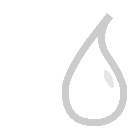# Laplacian nodeThis documentation is for version 4.0 of Laplacian (net.sf.cimg.CImgLaplacian).

## Description

Blur input stream, and subtract the result from the input image. This is not a mathematically correct Laplacian (which would be the sum of second derivatives over X and Y).

Uses the ‘vanvliet’ and ‘deriche’ functions from the CImg library.

CImg is a free, open-source library distributed under the CeCILL-C (close to the GNU LGPL) or CeCILL (compatible with the GNU GPL) licenses. It can be used in commercial applications (see http://cimg.eu).

Input

Description

Optional

Source

No

Yes

## Controls

Parameter / script name

Type

Default

Function

Size / `size`

Double

x: 3 y: 3

Size (diameter) of the filter kernel, in pixel units (>=0). The standard deviation of the corresponding Gaussian is size/2.4. No blur is applied if size < 0.24 (Gaussian and quasi-Gaussian) or <= 1 (box, triangle and quadratic).

Uniform / `uniform`

Boolean

Off

Apply the same amount of blur on X and Y.

Filter / `filter`

Choice

Gaussian

Bluring filter. The quasi-Gaussian filter should be appropriate in most cases. The Gaussian filter is more isotropic (its impulse response has rotational symmetry), but slower.
Quasi-Gaussian (quasigaussian): Quasi-Gaussian filter (0-order recursive Deriche filter, faster) - IIR (infinite support / impulsional response).
Gaussian (gaussian): Gaussian filter (Van Vliet recursive Gaussian filter, more isotropic, slower) - IIR (infinite support / impulsional response).
Box (box): Box filter - FIR (finite support / impulsional response).
Triangle (triangle): Triangle/tent filter - FIR (finite support / impulsional response).

(Un)premult / `premult`

Boolean

Off

Divide the image by the alpha channel before processing, and re-multiply it afterwards. Use if the input images are premultiplied.

Invert Mask / `maskInvert`

Boolean

Off

When checked, the effect is fully applied where the mask is 0.

Mix / `mix`

Double

1

Mix factor between the original and the transformed image.